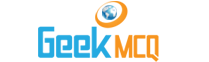# Entry Test MCQ :: Mixture Problems

1.  The percentage of water in 20 litres of adulterated milk is 10. The quantity of water to be added to it to increase the %age of water to 25% is
 A. 4 litres B. 5 litres C. 6 litres D. 7 litres

2.  In what proportion must a grocer mix rice at Rs: 1.2 per kg and Rs: 1.44 per kg so as to make a mixture worth Rs: 1.3 per kg?
 A. 18?24 B. 7?5 C. 3?4 D. 2?5

3.  In what proportion must a man mix milk at Rs: 11 per liter with milk at Rs: 6 per liter so the mixture be worth Rs: 8 per liter?
 A. 2?3 B. 3?2 C. 5?7 D. 5?6

4.  A mixture of 70 litres of milk and water contains 10% water. How much water must be added to make water 12
 A. 2 B. 5 C. 10 D. 12

5.  One liquid contains 20% of water another Contains 35% of water. A glass filled with 5 parts of first liquid and 10 parts of second the percentage of water in the new mixture
 A. 37% B. 30% C. 45% D. 20%

6.  If 4 Kg rice of superior quality of Rs: 6 a Kg be mixed with 6 kg. Rice at Rs: 4 per Kg of inferior quality the price of the mixture per kg shall be
 A. Rs: 5.00 B. Rs: 4.80 C. Rs: 4.70 D. Rs: 4.35

7.  The ratio in which the two quantities of rice at Rs: 6 and Rs: 4 per kg be mixed respectively in order the mixture may cost Rs: 4.80/kg is
 A. 2?3 B. 3?2 C. 1?4 D. 1?5

8.  A jar contains three parts of pure milk and one part of water. How much of the mixture must be drawn and water substituted in order that the result mixture may be half milk and half water
 A. 1/4 th B. 1/2nd C. 1/3rd D. 1/5th

9.  A shopkeeper mixes two kinds of sugar. One costing Rs: 3.50 kg and the other Rs: 2.25 Kg so that the price of the mixture is Rs: 2.75/kg. The ration of the first kind of the sugar to that of the second must be
 A. 1?2 B. 1?3 C. 2?3 D. None of these

10.  A producer blends two qualities of rice one costing Rs: 12.50 per kg and other costing Rs: 14:00 per kg in the ration of 4:5. Find the profit percent if he sells the mixture at the rate of Rs: 16 per kg
 A. 10% B. 15% C. 20% D. 25%